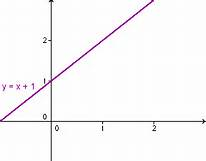# Basic Math for Statistics Courses

After a difficult past with mathematics, you find yourself in a a statistics 101 course. I am sure you were open-minded to the experience. But there are a few remnants of mathematics that tend to crop up every now and again. I will list a few things you may want to keep in mind.

1. Simplifying equations: Please remember to work on multiplication and division before addition and subtraction .(ex. 4x-55/11+5=0=> 4x-5+5=0 =>  4x=0)
2. Graphical equation: Please know how to plot a graph (ex.  y=x+1 which is really y=x with an intercept through y=1, x=0. You would graph it as follows3. Know how to calculate C(n,r) = n! / ( r! (n – r)! ) n=total sample  r=# of elements chosen. Typically seen in a binomial distribution probability calulation.
4. Know how to calculate exponentials and logs. Example: am+n = am * an
am-n = am / an
am*n = (am)n
(a * b)n = an * bn
(a / b)n = an / bn
a-n = 1 / an
a1 = a
a0 = 1
00 = 1
5. How to interpret decimals. If something is .0987 , you should be able to convert it to a percent, for example 9.87%
6. Greek symbols. There are a few greek symbols that show up in both math and statistics. Here is a short list:http://stattrek.com/statistics/notation.aspx

This is just a few examples..

If you enjoyed this blog, there are more to follows-Amy

This site uses Akismet to reduce spam. Learn how your comment data is processed.